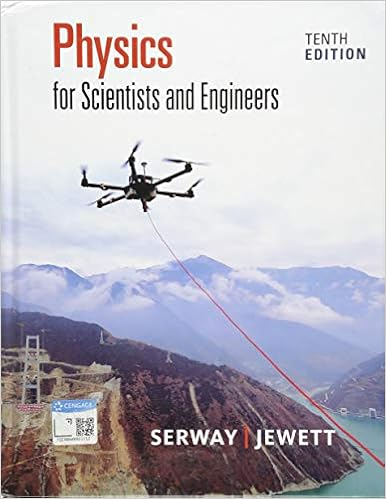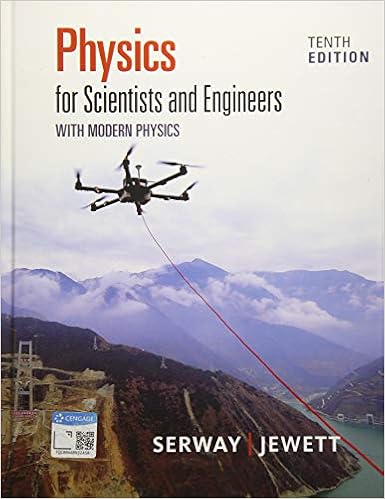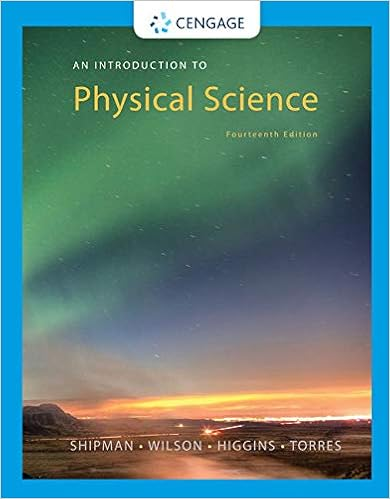# Question 6 go to the grid on page 3 put a dot

• Homework Help
• 5
• 25% (4) 1 out of 4 people found this document helpful

This preview shows page 5 out of 5 pages.

Question 6Go to the grid on page 3. Put a dot (representing a data point) at the intersection of 100% rock (x-axis) and 3.0 g/cm3(y-axis) to indicatea moon that is 100% rock. Likewise, put a dot at the intersection of 0% rock (x-axis) and 0.9 g/cm3to indicate a moon that is 100% ice.Plot the other points from the data table. Failure to plot ALL the points will result in points taken off. Draw a straight line from (0,0.9)and (100,3.0). USE A RULER. (Lines drawn without a ruler will result in loss of points.)Question 7Using the GRAPH on page 3, estimate the value of “% Rock” (the 4thand last column of table 1 on page 2). Write in the value of amoon’s percentage of rock (based on its density) in this table. To repeat: this value of each moon’s percentage of rock is read from thegraph. Note that a moon cannot be more than 100% rock (or ice).Question 9Beginning at the bottomof page 3 and continuing onto page 4 is a discussion of how to compare determining a moon’s percentage ofrock by two methods: graphical and algebraic. The moon chosen for the exercise is Callisto. From table 1 (top of page 2), you knowCallisto’s density—1.8 g/cm3—and have determined its percentage of rock (from the graph). Now, to determine Callisto’s percentageof rock algebraically, you use the first equation found just after the graph on page 3. However, this equation has two variables, whichmakes itawkward to work with. At the top of page 4, we have an equation with just one variable: “x.” But the equation given needssimplification. That is what you are to do in part (a): show the two steps needed to simplify the equation by writing them in the box. Inpart (b), youare going to solve the equation to determine Callisto’s percentage of rock. On the left side of the equation, you haveDmoon,which is 1.8 (you know that the moon is Callisto, and its density is found in table 1). The right side of the equation is the simplifiedversion worked out in part (a). Solve the equation for “x.” That will bethe percentage of rock of Callisto. Write that value into the blankspace for part (c). In part (d) compare the Callisto’s density determined graphically with the value just determined algebraically. Justplug the two numbers (graphical value and algebraic value) into the formula at the bottom of page 4 and calculate the answer.
##### We have textbook solutions for you!The document you are viewing contains questions related to this textbook.
Chapter 1 / Exercise 1
Physics for Scientists and Engineers
Jewett/SerwayExpert VerifiedBrowse all Textbook Solutions
End of preview. Want to read all 5 pages?

Course Hero member to access this document

Term
Spring
Professor
RichardRusso
Tags
Pluto, Dwarf planet
##### We have textbook solutions for you!
The document you are viewing contains questions related to this textbook.The document you are viewing contains questions related to this textbook.
Chapter 1 / Exercise 1
Physics for Scientists and Engineers
Jewett/SerwayExpert Verified
•••